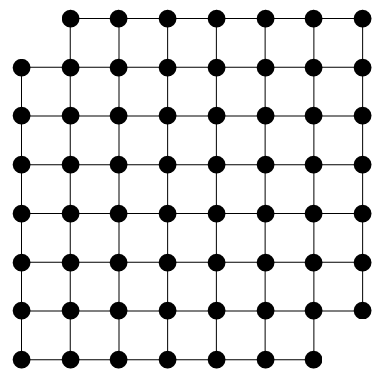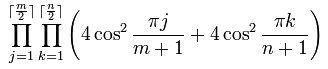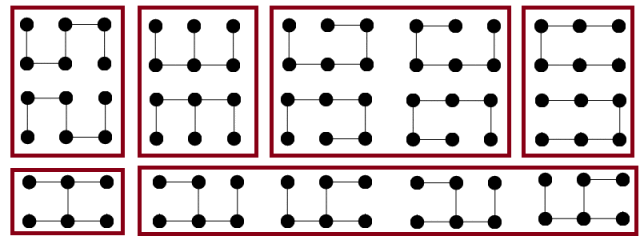# Permanent-determinant method

The determinant of a matrix can be regarded, most naturally, as the volume scaling factor of the corresponding linear map. However, one of the formulae for finding the determinant of a$n \times n$ square matrix is to compute$\sum_{\sigma \in S_n} sgn(\sigma) \prod_{i=1}^n A_{i,\sigma(i)}$, where we sum over all permutations and take their signs into account.

But what if we omit the term$sgn(\sigma)$? We get a related notion, known as the permanent of a matrix. This is far less useful than the determinant, but it does have a few applications.

### Perfect matchings

Suppose we have a bipartite graph, and want to compute the number of perfect matchings. In this case, we can express it as the permanent of the biadjacency matrix.One special family of bipartite graphs are the crown graphs. A crown graph can be defined as a bipartite graph, the biadjacency matrix of which is the complement of the identity matrix. The permanent of a crown graph on 2n vertices is given by the closest integer to$\dfrac{n!}{e}$.Crown graphs on 6, 8 and 10 vertices, respectively

The menage problem asks how many ways n couples (each containing one man and one woman; I apologise for the heteronormative nature of this) can be seated around a circular table of 2n seats, such that each person sits adjacent to neither his or her partner, nor anyone of the same same gender. It is not too difficult to see these arrangements correspond to Hamiltonian cycles of crown graphs. The solution sequence is given by A094047.

### Domino tilings

Another type of bipartite graph arises when you replace each square in a polyomino with a vertex, and join adjacent vertices with edges. For example, the bipartite graph arising from the Mutilated Chessboard Problem is shown below:Since its biadjacency matrix has 32 rows but only 30 columns, it is not square and therefore does not have a well-defined permanent. Hence, there is no way to tile the mutilated chessboard with dominoes.

It transpires that in the case of tiling polyominoes with dominoes, we can define a slightly altered biadjacency matrix, by weighting vertical edges with 1 and horizontal edges with the imaginary unit i. In that case, the determinant of the new matrix is equal to the permanent of the old one, and therefore the number of domino tilings.

This may seem like a pointless modification, but it massively accelerates computation. General determinants can be computed much more efficiently than permanents, namely in polynomial time$O(n^3)$. On the other hand, if arbitrary permanents can be calculated in polynomial time, then P = NP follows as a corollary.

For a$m \times n$ chessboard, the determinant of the modified biadjacency matrix was proved by Kasteleyn to have the following closed form:For m = 2, the number of tilings is the Fibonacci number F(n+1).

### Spanning trees

The number of spanning trees of a particular graph can also be expressed as the determinant of a matrix, thanks to Kirchoff’s theorem. It is a remarkable fact that the number of domino tilings of a$(2m - 1) \times (2n - 1)$ chessboard with one corner removed is precisely the number of spanning trees of a m by n grid graph, as mentioned here. Here are the 15 spanning trees of a particular grid graph, grouped into congruence classes:And here are the 15 corresponding domino tilings:I have joined pairs of tilings with an edge if they can be interconverted by rotating a single square of two dominoes. It has been proved that for any simply-connected polyomino, the resulting graph of domino tilings is connected. Of course, this is untrue for multiply-connected polyominoes (consider the two tilings of a 3 × 3 square with the centre removed).

In this case, the resulting graph of tilings is bipartite. This is true in general, as the number of horizontal dominoes (modulo 4) must alternate between 0 and 2 every time you move along an edge. Indeed, in the example shown above, the graph corresponds to a polyomino. This, however, is just a coincidence: with arbitrarily large polyominoes, we can get tilings with arbitrarily many neighbours.

This entry was posted in Uncategorized. Bookmark the permalink.

### 0 Responses to Permanent-determinant method

1.Volker Grabsch says:

Something went wrong with the last picture. In its original size, it looke fine:

https://cp4space.files.wordpress.com/2013/03/neighbours.png

However, the resized version (as shown in this blog) show a distorted image with vertical black and white stripes:

https://cp4space.files.wordpress.com/2013/03/neighbours.png?w=640&h=384

•apgoucher says:

Yes, WordPress messes up images occasionally. I don’t know how to remedy this, and it may be browser-dependent.

•wojowu says:

It gets weirder – soon after publication (very soon, I check blog very often :P) picture was resized, but it looked normal. Several hours later picture got distorted

2.Sam Cappleman-Lynes says:

Frustrating! I tentatively discovered yesterday the result about spanning trees on m by n grid graphs and domino tilings of a (2m-1) by (2n-1) board with a corner removed. I came up with a supposed bijection at the time, was able today to prove the correctness of said bijection, and was planning to submit it as an Olympiad problem. I Googled “spanning tree grid graph domino tiling” to check whether the result was known, before submitting it, and Google brought me here!

•apgoucher says:

It’s not particularly well-known; I only found out about it through the OEIS. What was your proposal? (Don’t say it here; we’ll transfer this discussion to the more secure place.)

•Sam Cappleman-Lynes says:

If you have the ability to delete/censor comments on here, that might be a good idea.